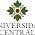## Wednesday, February 29, 2012

### XYZ geographic data interpolation, part 2

Having recently received a comment on a post regarding geographic xyz data interpolation, I decided to return to my original "xyz.map" function and open it up for easier interpretation. This should make the method easier to adapt and follow.

The above graph shows the distance to Mecca as interpolated from 1000 randomly generated lat/lon data using the "interp" function of the akima package. Several functions, found within this blog, are needed to reproduce the plot (pos2coord, earth.dist, new.lon.lat, color.palette, val2col, image.scale). One thing you will notice is the strip of uninterpolated area within the stereographic projection. This is a problem that I have yet to resolve and has to do with the fact that the interpolation is not considering the connection along the 180° longitude line. This will probably require some other type of interpolation based on geographic distances rather than Cartesian coordinates.

R code to produce the above graph...

```#required packages
require(akima)
require(maps)
require(mapproj)

source("pos2coord.R")
source("earth.dist.R")
source("new.lon.lat.R")
source("color.palette.R")
source("val2col.R")
source("image.scale.R")

###create xyz data
set.seed(1)
mecca <- data.frame(lon=39.826138, lat=21.422508)
x <- runif(10000, min=-180, max=180)
y <- runif(10000, min=-90, max=90)
z <- earth.dist(x, y, mecca\$lon, mecca\$lat)
xyz_data <- cbind(x,y,z)

###interpolate data
INTERP <- interp(x, y, z, xo=seq(-180, 180, length = 100), yo=seq(-90, 90, length = 100))

###basic plot
image(INTERP, col=rainbow(100))

###projection plots
#make polygons
polys<-vector("list", length(as.vector(INTERP\$z)))
for(i in 1:length(polys)){
lonx <- pos2coord(pos=i, dim.mat=dim(INTERP\$z))
laty <- pos2coord(pos=i, dim.mat=dim(INTERP\$z))
ifelse(laty < length(INTERP\$y), neigh_y<-c(laty+1,lonx), neigh_y<-c(laty-1,lonx))
ifelse(lonx < length(INTERP\$x), neigh_x<-c(laty,lonx+1), neigh_x<-c(laty,lonx-1))
dist_y <- earth.dist(INTERP\$x[lonx], INTERP\$y[laty], INTERP\$x[neigh_y], INTERP\$y[neigh_y])
dist_x <- earth.dist(INTERP\$x[lonx], INTERP\$y[laty], INTERP\$x[neigh_x], INTERP\$y[neigh_x])
s1 = new.lon.lat(INTERP\$x[lonx], INTERP\$y[laty], 180, dist_y/2)
s3 = new.lon.lat(INTERP\$x[lonx], INTERP\$y[laty], 0, dist_y/2)
s2 = new.lon.lat(INTERP\$x[lonx], INTERP\$y[laty], 270, dist_x/2)
s4 = new.lon.lat(INTERP\$x[lonx], INTERP\$y[laty], 90, dist_x/2)
polys[[i]] = cbind(c(s2, s2, s4, s4), c(s1, s3, s3, s1))
}

#values to colors
pal <- color.palette(rev(c("purple","black","red","yellow")), n.steps.between=c(2,5,20))
COL <- val2col(INTERP\$z, col=pal(100))

#plot parameters
WIDTHS=c(4,4,1)
HEIGHTS=c(4)
OMI=c(0.1,0.1,0.1,0.1)

#define plot device and layout
png("xyz_2_projections.png", width=sum(WIDTHS)+sum(OMI[c(2,4)]), height=sum(HEIGHTS)+sum(OMI[c(1,3)]), units="in",res=400)
#x11(width=sum(WIDTHS)+sum(OMI[c(2,4)]), height=sum(HEIGHTS)+sum(OMI[c(1,3)]))
layout(matrix(c(1:3),nrow=1,ncol=3), width=WIDTHS, height=HEIGHTS, respect=TRUE)
layout.show(3)

#1st plot - perspective projection
XLIM=c(-180,180)
YLIM=c(-90,90)
PROJ="perspective"
ORIENT=c(mecca\$lat,mecca\$lon,0)
PAR=2
par(mai=c(0.1,0.1,0.5,0.1))
map("world", projection=PROJ, orientation=ORIENT, par=PAR, xlim=XLIM, ylim=YLIM, col=NA)
for(i in 1:length(polys)){
polygon(mapproject(x=polys[[i]][,1], y=polys[[i]][,2]), col=COL[i], border=COL[i], lwd=0.1)
}
map("world", add=TRUE, projection="", xlim=XLIM, ylim=YLIM, col="grey")
map.grid(c(-180, 180, -80, 80), nx=12, ny=6, labels=FALSE, col="grey")
mtext("Perspective projection", side=3, line=1)

#2nd plot - stereographic projection
XLIM=c(-180,180)
YLIM=c(-90,90)
PROJ="stereographic"
ORIENT=c(mecca\$lat,mecca\$lon,0)
PAR=NULL
par(mai=c(0.1,0.1,0.5,0.1))
map("world", projection=PROJ, orientation=ORIENT, par=PAR, xlim=XLIM, ylim=YLIM, col=NA)
for(i in 1:length(polys)){
polygon(mapproject(x=polys[[i]][,1], y=polys[[i]][,2]), col=COL[i], border=COL[i], lwd=0.1)
}
map("world", add=TRUE, projection="", xlim=XLIM, ylim=YLIM, col="grey")
map.grid(c(-180, 180, -80, 80), nx=12, ny=6, labels=FALSE, col="grey")
mtext("Stereographic projection", side=3, line=1)

#3rd plot - color scale
par(mai=c(0.1,0.1,0.5,0.7))
image.scale(INTERP\$z, col=pal(100), horiz=FALSE, xaxt="n", yaxt="n", xlab="", ylab="")
box()
axis(4)
mtext("Distance to Mecca [km]", side=4, line=3)

#close device
dev.off()```
Created by Pretty R at inside-R.org

1.The projection function failed for some input data.

2.Yes, I think for some projections, the country polygons do not always plot perfectly. However, in this example, you do not really see a dramatic effect. I have come across some tricks to avoid these problems in other blogs - will try to address this at some point in the future.

3.I obtained the right result! Thank you very much. :)

4.I like the hole at the 180th meridian. Yes, it is a data artifact, but does provide a nice guide to where things are on the map.

5.Well, there's that ... :-)
You can also add labels to the grid lines in the map.grid function.

6.in:
#define plot device and layout
the first code line(png)failed,why?

7.I obtained this error:
In map("world", projection = PROJ, orientation = ORIENT, par = PAR, :
projection failed for some data

waht could be the reason?.Thanks

8.Hi ANGEL666 - As I mentioned in an earlier comment, some country polygons might be cut off or not plotted correctly by some projections. You don't see too much of a noticeable affect here though. I'm not sure why your "png" line failed. Was there a warning? Maybe, make sure that you have closed all open devices before starting the plot next time (by running "dev.off()" until no devices remain). - Marc